# Fundamental solution

of a linear partial differential equation

A solution of a partial differential equation,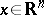, with coefficients of class, in the form of a function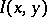that satisfies, for fixed, the equationwhich is interpreted in the sense of the theory of generalized functions, whereis the delta-function. There is a fundamental solution for every partial differential equation with constant coefficients, and also for arbitrary elliptic equations. For example, for the elliptic equation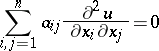with constant coefficients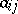forming a positive-definite matrix, a fundamental solution is provided by the function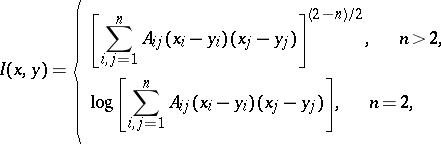where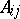is the cofactor ofin the matrix.

Fundamental solutions are widely used in the study of boundary value problems for elliptic equations.